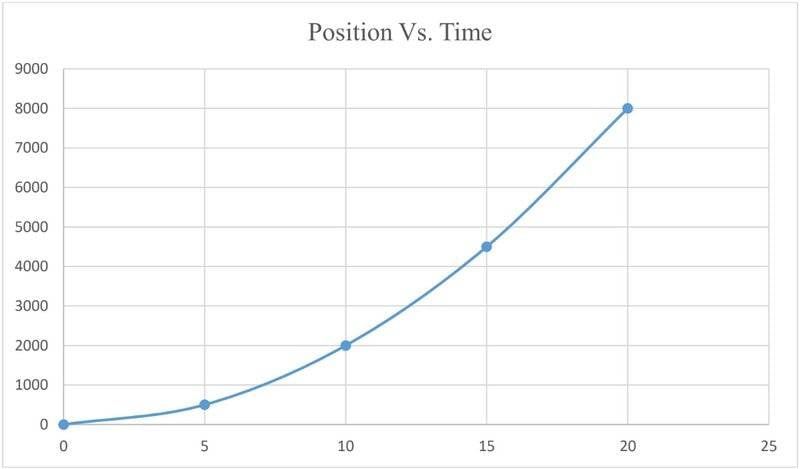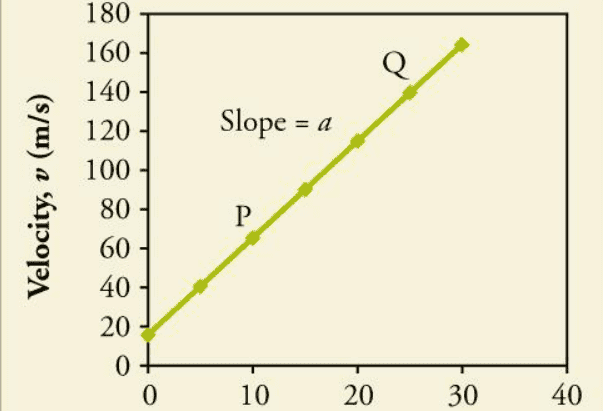# Velocity and acceleration graphs

• bbsgirl10
The points on the provided position versus time graph are very nicely patterned. The five y coordinates are at 0 hash marks, 0.5 hash marks, 2.0 hash marks, 4.5 hash marks and 8.0 hash marks. Double each of them and that is 0, 1, 4, 9, 16. It does not get much more obvious than that.

#### bbsgirl10

Homework Statement
.
Relevant Equations
nilIt doesn’t feel like you posted the entire question, but maybe all you left out was "Find".
How would you go about finding the initial velocity and acceleration from the position/time graph?
But to answer your question, no, the two graphs represent different motions.

Last edited:
haruspex said:
It doesn’t feel like you posted the entire question, but maybe all you left out was "Find".
How would you go about find the initial velocity and acceleration from the position/time graph?
But to answer your question, no, the two graphs represent different motions.
The graph is just an example to show the type of motion it travels. I just want to know whether the acceleration and initial velocity will be same for both position/time graph and Velocity time graph

bbsgirl10 said:
The graph is just an example to show the type of motion it travels. I just want to know whether the acceleration and initial velocity will be same for both position/time graph and Velocity time graph
And I answered that (from the data in these graphs) they will not be.

Last edited:
haruspex said:
And I answered that they will not be.
may i know why? because i get around the same value for acceleration and initial velocity based on experiment I got a = 0.200 (for postion/time graph) and a =0.203 (for velocity/time graph). I also get Initial velocity = 0.0711 (for postion/time graph) and Initial velocity = 0.0811 (for velocity/time graph)

If you've both ##d:t## and ##v:t## graphs representing the same experiment, they will both show the same thing regarding velocity. (The two graphs you give as an example are not for the same experiment).

Of course, you can't get initial displacement from a velocity graph.

Last edited:
•bbsgirl10
bbsgirl10 said:
may i know why? because i get around the same value for acceleration and initial velocity based on experiment I got a = 0.200 (for postion/time graph) and a =0.203 (for velocity/time graph). I also get Initial velocity = 0.0711 (for postion/time graph) and Initial velocity = 0.0811 (for velocity/time graph)
I think I misunderstood your post 3. See my edited post #4. (I don't see why you posted those two graphs with the question - it's just confusing.)
Yes, if you are looking at such graphs from the same experiment, which the graphs you posted are not, then of course you should in principle get the same answers to whatever questions you pose. But it might be quite difficult to extract accurate values in some cases.
For the initial velocity, that is very difficult to read off the s/t graph directly. Since you are assuming acceleration is constant you could pick three data points and solve the quadratic. That will give the acceleration too.

bbsgirl10 said:
may i know why? because i get around the same value for acceleration and initial velocity based on experiment I got a = 0.200 (for postion/time graph) and a =0.203 (for velocity/time graph). I also get Initial velocity = 0.0711 (for postion/time graph) and Initial velocity = 0.0811 (for velocity/time graph)
Welcome!
Could you explain your experiment and collected data a little more?
Acceleration is independent from any single value of velocity, initial or final.
A car can accelerate at the same constant rate from 50 to 60 mph, as from 20 to 30 mph.

Assuming uniform acceleration for the top graph, then solving x = ½at2 for a , using the point (20,8000) gives a=40 (units unknown).
Eyeballing the bottom graph for P(10,65) and Q(25,140) then calculating slope gives a=5m/s2 , so not the same experimental data as in the top graph.
bbsgirl10 said:
Homework Statement:: .
Relevant Equations:: nil

View attachment 314087View attachment 314088

cormsby said:
Assuming uniform acceleration for the top graph, then solving x = ½at2 for a , using the point (20,8000) gives a=40 (units unknown).
Eyeballing the bottom graph for P(10,65) and Q(25,140) then calculating slope gives a=5m/s2 , so not the same experimental data as in the top graph.
If you read through the thread you will find the posted graphs are irrelevant to the question being asked. Ignore them.

haruspex said:
Since you are assuming acceleration is constant you could pick three data points and solve the quadratic. That will give the acceleration too.
The points on the provided position versus time graph are very nicely patterned. The five y coordinates are at 0 hash marks, 0.5 hash marks, 2.0 hash marks, 4.5 hash marks and 8.0 hash marks. Double each of them and that is 0, 1, 4, 9, 16. It does not get much more obvious than that.

An exact five point fit to a quadratic model where the data points all hit hash marks on round numbers. That makes it likely that the data was fudged by an instructor rather than measured from an experiment.

We seem to have a parabola with its apex at (0, 0). The conclusion is that the initial velocity for that particular position versus time graph is zero.

jbriggs444 said:
The points on the provided position versus time graph are very nicely patterned.
Yes, but, as has been discovered in the course of this thread, the posted graphs are irrelevant and should be ignored. We do not have the actual data that gave rise to the question.

•jbriggs444
Almost every single poster noticed that the graphs are for different motions... but, nobody noticed that everybody else did.

Amazing we get anything done here, at all.

••256bits, erobz and jbriggs444
cormsby said:
But that is a misreading, which I also made. @bbsgirl is asking whether in general, given a velocity time graph and a distance time graph for the same motion (which those graphs are not) the accelerations deduced from them would be the same. If you look at the numbers quoted in those posts they clearly do not correspond to either posted graph, but do closely correspond to each other.

haruspex said:
@bbsgirl is asking whether in general
I did not see the phrase "in general", and I think you did not, either.
Perhaps we should let bbsgirl explain herself, rather than speculate.

cormsby said:
I did not see the phrase "in general", and I think you did not, either.
Perhaps we should let bbsgirl explain herself, rather than speculate.
My original response to @bbsgirl10:
haruspex said:
the two graphs represent different motions.
to which she replied:
bbsgirl10 said:
The graph is just an example to show the type of motion it travels.
bbsgirl10 said:
i get around the same value for acceleration and initial velocity based on experiment I got a = 0.200 (for postion/time graph) and a =0.203 (for velocity/time graph). I also get Initial velocity = 0.0711 (for postion/time graph) and Initial velocity = 0.0811 (for velocity/time graph)
From those data it is clear she is not using the posted graphs in her calculations. She never did post the data she was using and, unfortunately, appears to have abandoned the thread. So you can go on believing the graphs are relevant to the question but no other responder does.

•SammyS
haruspex said:
you can go on believing
I am not a "believer".
I proceed upon what I see, and what I know.
I recommend everyone else do the same.